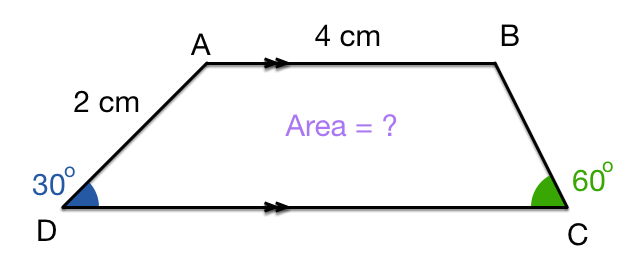# Breaking A Trapezium Apart

Geometry Level 2In the figure above, $AB$ is parallel to $CD$. If $AB =4 \text{ cm}$ and $AD=2 \text{ cm}$, angles $D$ and $C$ are $30^\circ$ and $60^\circ$ respectively. Find the area of the figure (in $\text{cm}^2$).